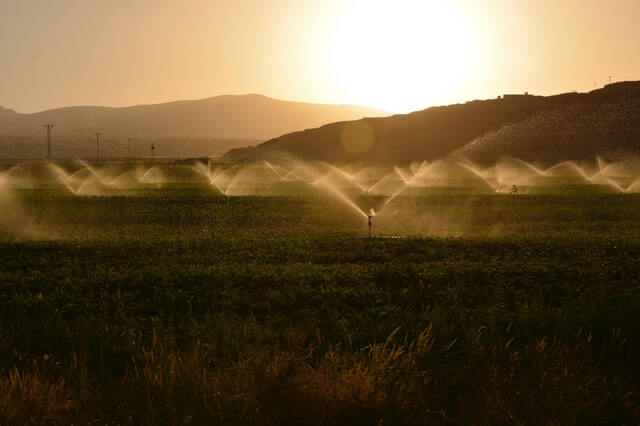# What is Irrigation Efficiency? Different Types of Irrigation Efficiency

2nd September 2020

## What is Irrigation Efficiency?

The amount of supplied water is not fully utilised for the growth of crops because there are several reasons for water loss. Irrigation efficiency is defined as the ratio of the amount of water available to the amount of water supplied from the reservoir. It is expressed in percentage.

### Different Types of Irrigation Efficiency

Following are the different types of irrigation efficiency:

#### 1. Efficiency of Water Conveyance

It is the ratio of the amount of irrigation water that is available at the outlets, to the amount of water supplied from the reservoir. It may be symbolised by ηc. It can be obtained by the following expression.$\eta_{c} = \frac{W_{l}}{W_{r}} \times 100$

Where,

• ηc = Efficiency of water conveyance.
• Wl = Amount of water available at the outlet’s point.
• Wr = Amount of water supplied from the reservoir.

#### 2. Efficiency of Water application

It is the ratio of the amount of water stored in the root zone of plants to the amount of water supplied at the outlet point of the field. It may be symbolised by ηa. It can be obtained by the following expression.$\eta_{a} = \frac{W_{z}}{W_{l}} \times 100$

Where,

• ηa = Water application efficiency
• Wz = Amount of water stored in the root zone.
• Wl = Amount of water supplied at the outlet point of the field.

#### 3. Efficiency of Water use

It is the ratio of the amount of water beneficially used to the amount of water applied. It may be expressed by ηu. It can be obtained by the following expression.$\eta_{u} = \frac{W_{u}}{W_{l}} \times 100$

Where,

• ηu = Efficiency of water use
• Wu = Water used
• Wl = Water Applied

#### 4. Efficiency of Consumptive use

It is the ratio of the amount of consumptive use of water to the amount of water depleted from the root zone. It is expressed by ηcu. It can be obtained by the following expression.$\eta_{cu} = \frac{C_{u}}{W_{p}} \times 100$

Where,

• ηcu = Efficiency of Consumptive.
• Cu = Consumptive use of water.
• Wp = Amount of water depleted from root zone.